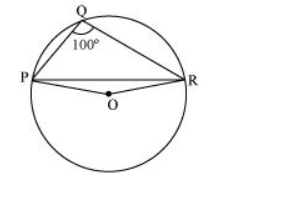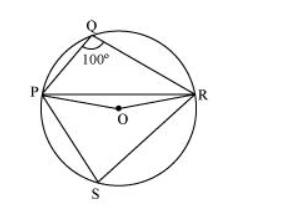# In the given figure, ∠PQR = 100°,

Question. In the given figure, $\angle P Q R=100^{\circ}$, where $P, Q$ and $R$ are points on a circle with centre $O$. Find $\angle O P R$.Solution:Consider PR as a chord of the circle.

Take any point S on the major arc of the circle.

$\angle P Q R+\angle P S R=180^{\circ}$ (Opposite angles of a cyclic quadrilateral)

$\Rightarrow \angle \mathrm{PSR}=180^{\circ}-100^{\circ}=80^{\circ}$

We know that the angle subtended by an arc at the centre is double the angle subtended by it at any point on the remaining part of the circle.

$\therefore \angle \mathrm{POR}=2 \angle \mathrm{PSR}=2\left(80^{\circ}\right)=160^{\circ}$

In $\triangle \mathrm{POR}$,

OP = OR (Radii of the same circle)

$\therefore \angle O P R=\angle O R P($ Angles opposite to equal sides of a triangle $)$

$\angle O P R+\angle O R P+\angle P O R=180^{\circ}$ (Angle sum property of a triangle)

$2 \angle O P R+160^{\circ}=180^{\circ}$

$2 \angle O P R=180^{\circ}-160^{\circ}=20^{\circ}$

$\angle O P R=10^{\circ}$